### Home > A2C > Chapter 8 > Lesson 8.1.2 > Problem8-28

8-28.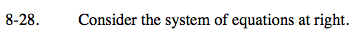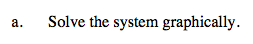The first equation is a circle with radius 5 and center (0, 0).

The second equation is a parabola with vertex (0, 3).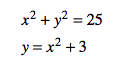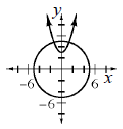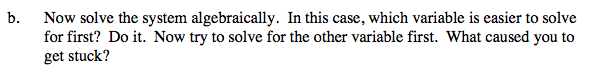What happens when you substitute for y in the first equation?

Try solving for x2 in the second equation and substituting that into the first equation.